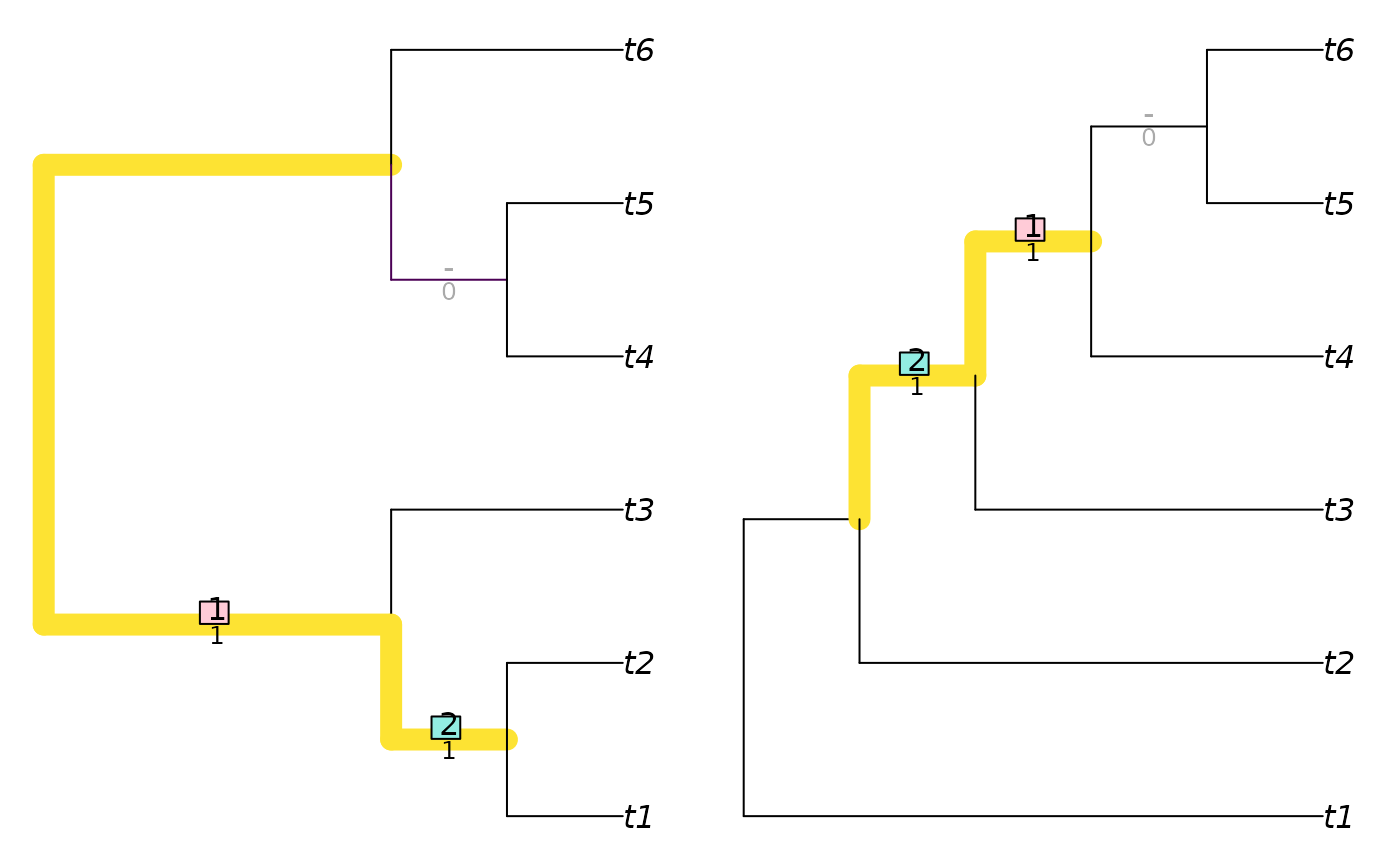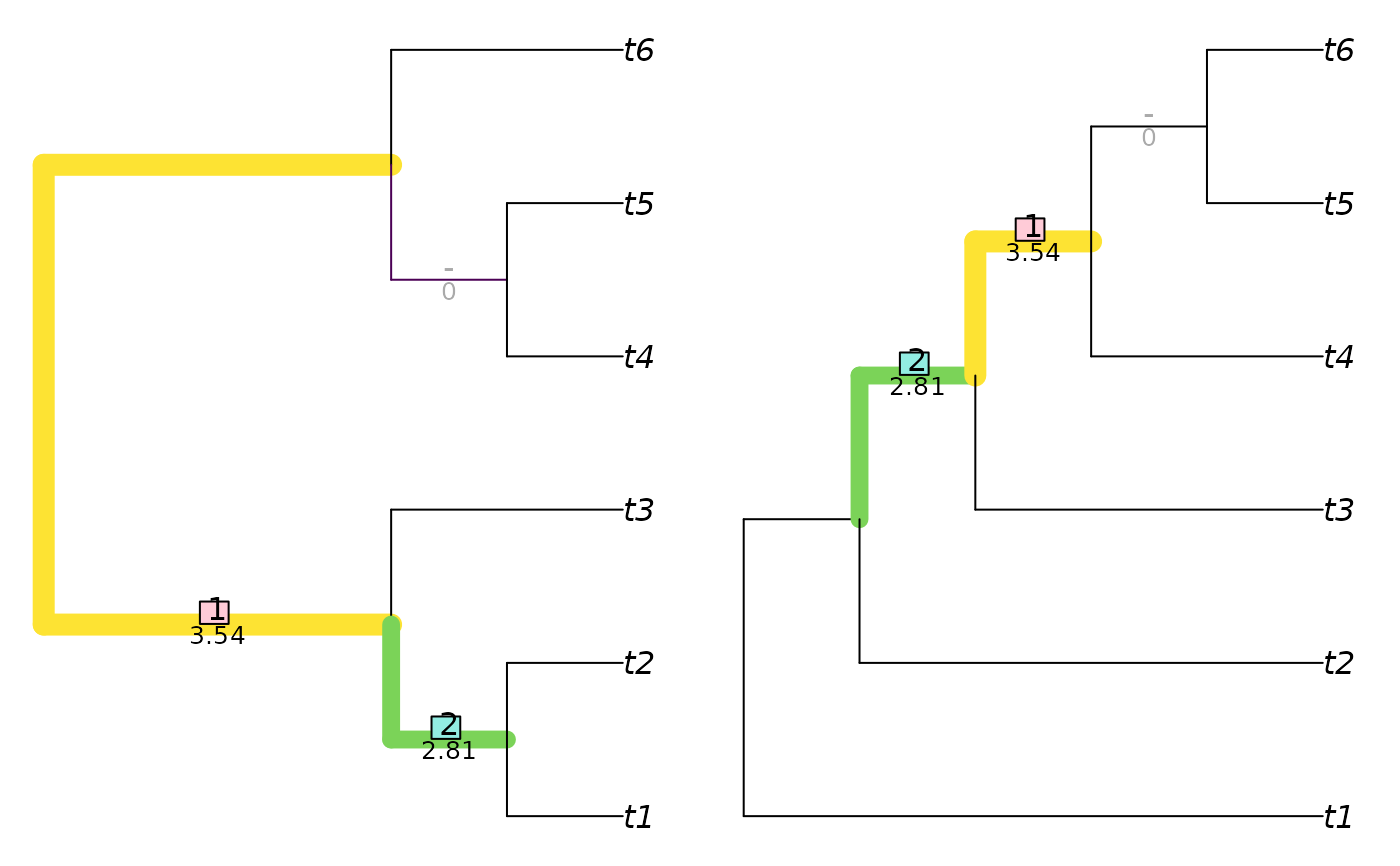Depict the splits that are matched between two trees using a specified Generalized Robinson–Foulds similarity measure.

VisualizeMatching(
Func,
tree1,
tree2,
setPar = TRUE,
precision = 3L,
Plot = plot.phylo,
matchZeros = TRUE,
plainEdges = FALSE,
edge.width = 1,
edge.color = "black",
...
)

## Arguments

Func

Function used to construct tree similarity.

tree1, tree2

Trees of class phylo, with identical leaf labels.

setPar

Logical specifying whether graphical parameters should be set to display trees side by side.

precision

Integer specifying number of significant figures to display when reporting matching scores.

Plot

Function to use to plot trees.

matchZeros

Logical specifying whether to pair splits with zero similarity (TRUE), or leave them unpaired (FALSE).

plainEdges

Logical specifying whether to plot edges with a uniform width and colour (TRUE), or whether to draw edge widths according to the similarity of the associated splits (FALSE).

edge.width, edge.color, ...

Additional parameters to send to Plot().

## Details

Note that when visualizing a Robinson–Foulds distance (using Func = RobinsonFouldsMatching), matched splits are assigned a similarity score of 1, which is deducted from the total number of splits to calculate the Robinson–Foulds distance. Unmatched splits thus contribute one to total tree distance.

## Examples

tree1 <- TreeTools::BalancedTree(6)
tree2 <- TreeTools::PectinateTree(6)

VisualizeMatching(RobinsonFouldsMatching, tree1, tree2)VisualizeMatching(SharedPhylogeneticInfo, tree1, tree2, matchZeros = FALSE)